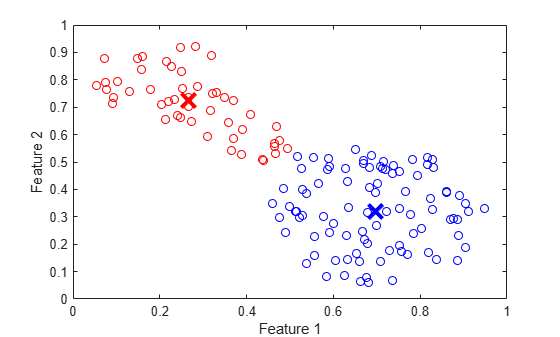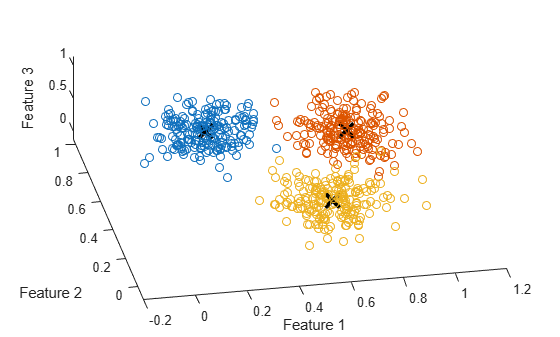# fcm

Fuzzy c-means clustering

## Syntax

``````[centers,U] = fcm(data)``````
``````[centers,U] = fcm(data,options)``````
``````[centers,U,objFcn] = fcm(___)``````
``````[centers,U,objFcn,info] = fcm(___)``````

## Description

``````[centers,U] = fcm(data)``` computes cluster centers (`centers`) and a fuzzy partition matrix (`U`) using default clustering options.By default, `fcm` clusters the data ten times, varying the number of clusters from 2 through 11.```

example

``````[centers,U] = fcm(data,options)``` specifies clustering options, such as the number of clusters and the distance metric.```

example

``````[centers,U,objFcn] = fcm(___)``` returns the objective function values at each optimization iteration for the optimal number of clusters.```

example

``````[centers,U,objFcn,info] = fcm(___)``` returns the clustering results for all numbers of clusters used along with the validity index used for determining the optimal number of clusters. When the distance metric specified in `options` is either ``` "mahalanobis"``` or `"fmle"`, `info` also contains the covariance matrices generated for each number of clusters.```

## Examples

collapse all

Load the data to cluster. Each row of `fcmdata` contains one data point. The two columns of `fcmdata` contain the feature values for each data point.

`load fcmdata.dat`

Specify clustering options using an `fcmOptions` object. For this example, set the number of clusters to 2 and use default values for the other options.

`options = fcmOptions(NumClusters=2);`

Find the cluster centers using fuzzy c-means clustering.

`[centers,U] = fcm(fcmdata,options);`
```Iteration count = 1, obj. fcn = 8.97048 Iteration count = 2, obj. fcn = 7.1974 Iteration count = 3, obj. fcn = 6.32558 Iteration count = 4, obj. fcn = 4.58614 Iteration count = 5, obj. fcn = 3.89311 Iteration count = 6, obj. fcn = 3.8108 Iteration count = 7, obj. fcn = 3.7998 Iteration count = 8, obj. fcn = 3.79786 Iteration count = 9, obj. fcn = 3.79751 Iteration count = 10, obj. fcn = 3.79744 Iteration count = 11, obj. fcn = 3.79743 Iteration count = 12, obj. fcn = 3.79743 Minimum improvement reached. ```

Classify each data point into the cluster with the largest membership value.

```maxU = max(U); index1 = find(U(1,:) == maxU); index2 = find(U(2,:) == maxU);```

Plot the clustered data and cluster centers.

```plot(fcmdata(index1,1),fcmdata(index1,2),"ob") hold on plot(fcmdata(index2,1),fcmdata(index2,2),"or") plot(centers(1,1),centers(1,2),"xb",MarkerSize=15,LineWidth=3) plot(centers(2,1),centers(2,2),"xr",MarkerSize=15,LineWidth=3) xlabel("Feature 1") ylabel("Feature 2") hold off```Create a random data set.

`data = rand(100,2);`

Specify the following FCM clustering options.

• Compute two clusters.

• To increase the amount of fuzzy overlap between the clusters, specify a large fuzzy partition matrix exponent.

• Suppress the command-window display of the objective function values for each iteration.

```options = fcmOptions(... NumClusters=2,... Exponent=3.0,... Verbose=false);```

Cluster the data.

`[centers,U] = fcm(data,options);`

`load clusterDemo.dat`

Configure an options object for computing three clusters and suppress the command-window output of the objective function values. Also, set the clustering termination conditions such that the optimization stops when either of the following occurs:

• The number of iterations reaches a maximum of 50.

• The objective function improves by less than 0.001 between two consecutive iterations.

```options = fcmOptions(... NumClusters=3,... MaxNumIteration=50,... MinImprovement=0.001,... Verbose=false);```

Cluster the data.

`[centers,U,objFun] = fcm(clusterDemo,options);`

The length of the objective function vector is less than 50; therefore the clustering did not reach the maximum number of iterations.

View the final three values of the objective function vector.

`objFun(end-2:end)`
```ans = 3×1 15.4353 15.4306 15.4305 ```

The optimization stopped because the objective function improved by less than 0.001 between the final two iterations.

`load clusterDemo.dat`

Specify the clustering options. For this example, cluster the data three times, once each for 2, 3, and 4 clusters. Suppress the command-window output.

```options = fcmOptions(... NumClusters=[2 3 4],... Verbose=false);```

Cluster the data. The results returned in `centers`, `U`, and `objFun` correspond to the optimal number of clusters. The results for all cluster counts are returned in `info`.

`[centers,U,objFun,info] = fcm(clusterDemo,options);`

View the optimal number of clusters.

`Nc = info.OptimalNumClusters`
```Nc = 3 ```

Verify the optimal number of clusters using the validity index values, which correspond to the cluster counts specified using `NumClusters`. The optimal number of clusters corresponds to the smallest validity index.

`info.ValidityIndex`
```ans = 1×3 0.3258 0.1891 5.1597 ```

The smallest validity index corresponds to a cluster count of 3.

Plot the clustered data using the optimal clustering results. First classify each data point into the cluster with the largest membership value.

```maxU = max(U); index1 = find(U(1,:) == maxU); index2 = find(U(2,:) == maxU); index3 = find(U(3,:) == maxU);```

Plot the clustered data and cluster centers.

```figure hold on scatter3(clusterDemo(index1,1),clusterDemo(index1,2),... clusterDemo(index1,3)) scatter3(clusterDemo(index2,1),clusterDemo(index2,2),... clusterDemo(index2,3)) scatter3(clusterDemo(index3,1),clusterDemo(index3,2),... clusterDemo(index3,3)) plot3(centers(:,1),centers(:,2),centers(:,3), ... "xk",MarkerSize=15,LineWidth=3) xlabel("Feature 1") ylabel("Feature 2") zlabel("Feature 3") view([-11 63]) hold off````load clusterDemo.dat`

Estimate the number of clusters (`Nc`) and the initial cluster centers (`initCenters`). For this example, use the `subclust` function.

```initCenters = subclust(clusterDemo,0.5); Nc = size(initCenters,1);```

Configure the FCM clustering to use the initial cluster centers as a starting point.

```options = fcmOptions(... NumClusters=Nc, ... ClusterCenters=initCenters);```

Cluster the data.

`[centers,U] = fcm(clusterDemo,options);`
```Iteration count = 1, obj. fcn = 103.934 Iteration count = 2, obj. fcn = 15.7792 Iteration count = 3, obj. fcn = 15.7792 Minimum improvement reached. ```

With initial estimates of the cluster centers, the FCM algorithm converges quickly.

## Input Arguments

collapse all

Data set to be clustered, specified as a matrix with Nd rows, where Nd is the number of data points. The number of columns in `data` is equal to the data dimensionality, that is, the number of features in each data point.

Clustering options, specified as an `fcmOptions` object.

## Output Arguments

collapse all

Final cluster centers, returned as a matrix with Nc rows containing the coordinates of each cluster center, where Nc is the number of clusters. The number of columns in `centers` is equal to the dimensionality of the data being clustered.

When `options.NumClusters` is a:

• Vector or `"auto"`, Nc is the optimal number of clusters.

• Scalar, Nc is equal to `options.NumClusters`.

Fuzzy partition matrix, returned as an Nc-by-Nd matrix. Element `U(i,j)` indicates the degree of membership μij of the jth data point in the ith cluster. For a given data point, the sum of the membership values for all clusters is one.

When `options.NumClusters` is a vector or `"auto"`, `U` corresponds to the optimal number of clusters.

Objective function values for each clustering iteration, returned as a vector with length equal to the number of clustering iterations.

When `options.NumClusters` is a vector or `"auto"`, `objFcn` corresponds to the optimal number of clusters.

Since R2023b

Detailed clustering results, returned as a structure with the following fields.

Number of clusters, as specified using `options.NumClusters`, returned as an integer or a vector of integers.

Cluster centers, returned as a cell array with length equal to the length of `NumClusters`. The cluster centers for the optimal number of clusters are returned in `centers`.

Each entry in `ClusterCenters` is a matrix with Nc rows, where Nc is the corresponding number of clusters returned in `NumClusters`.

Fuzzy partition matrices, returned as a cell array with length equal to the length of `NumClusters`. Each entry in `FuzzyPartitionMatrix` is a matrix with Nc rows, where Nc is the corresponding number of clusters returned in `NumClusters`.

The partition matrix for the optimal number of clusters is returned in `U`.

Objective function values, returned as a cell array with length equal to the length of `NumClusters`. Each entry in `ObjectiveFunctionValue` is a matrix with number of rows equal to the number of clustering iterations performed for the corresponding number of clusters returned in `NumClusters`.

The objective function values for the optimal number of clusters are returned in `objFcn`.

Covariance matrices, returned as a cell array with length equal to the length of `NumClusters`.

This field is returned when `options.DistanceMetric` is either `"mahalanobis"` or `"fmle"`.

Validity index values, returned as a scalar or a vector with the same length as `NumClusters`. The minimum validity index corresponds to the optimal number of clusters. 

Optimal number of clusters, returned as an integer. The optimal number of clusters is the value from `NumClusters` that corresponds to the minimum validity index in `ValidityIndex`.

The values returned in `centers`, `U`, and `objFcn` correspond to the optimal number of clusters.

## Tips

• To generate a fuzzy inference system using FCM clustering, use the `genfis` function. For example, suppose that you cluster your data using the following syntax.

`[centers,U] = fcm(data,fcmOpt);`

The first `M` columns of `data` correspond to input variables and the remaining columns correspond to output variables.

You can generate a fuzzy system using the same training data and FCM clustering configuration. To do so:

1. Configure the clustering options.

```opt = genfisOptions("FCMClustering"); opt.NumClusters = fcmOpt.NumClusters; opt.Exponent = fcmOpt.Exponent; opt.MaxNumIteration = fcmOpt.MaxNumIteration; opt.MinImprovement = fcmOpt.MinImprovement; opt.DistanceMetric = fcmOpt.DistanceMetric; opt.Verbose = fcmOpt.Verbose; ```
2. Extract the input and output variable data.

```inputData = data(:,1:M); outputData = data(:,M+1:end); ```
3. Generate the FIS structure.

`fis = genfis(inputData,outputData,opt);`

The fuzzy system `fis` contains one fuzzy rule for each cluster, and each input and output variable has one membership function per cluster. For more information, see `genfis` and `genfisOptions`.

## Algorithms

FCM is a clustering method that allows each data point to belong to multiple clusters with varying degrees of membership. To configure clustering options, create an `fcmOptions` object.

The FCM algorithm computes cluster centers and membership values to minimize the following objective function.

`${J}_{m}=\sum _{i=1}^{C}\sum _{j=1}^{N}{\mu }_{ij}^{m}{D}_{ij}^{2}$`

Here:

• N is the number of data points.

• C is the number of clusters. To specify this value, use the `NumClusters` option.

• m is fuzzy partition matrix exponent for controlling the degree of fuzzy overlap, with m > 1. Fuzzy overlap refers to how fuzzy the boundaries between clusters are, that is, the number of data points that have significant membership in more than one cluster. To specify the fuzzy partition matrix exponent, use the `Exponent` option.

• Dij is the distance from the jth data point to the ith cluster.

• μij is the degree of membership of the jth data point in the ith cluster. For a given data point, the sum of the membership values for all clusters is one.

The `fcm` function supports three types of FCM clustering:

• Classical FCM 

• Gustafson-Kessel FCM 

• Gath-Geva FCM 

These methods differ in the distance metric used for computing Dij. For more information, see Fuzzy Clustering.

 Bezdek, James C. Pattern Recognition with Fuzzy Objective Function Algorithms. Boston, MA: Springer US, 1981. https://doi.org/10.1007/978-1-4757-0450-1.

 Gustafson, Donald, and William Kessel. “Fuzzy Clustering with a Fuzzy Covariance Matrix.” In 1978 IEEE Conference on Decision and Control Including the 17th Symposium on Adaptive Processes, 761–66. San Diego, CA, USA: IEEE, 1978. https://doi.org/10.1109/CDC.1978.268028.

 Gath, I., and A.B. Geva. “Unsupervised Optimal Fuzzy Clustering.” IEEE Transactions on Pattern Analysis and Machine Intelligence 11, no. 7 (July 1989): 773–80. https://doi.org/10.1109/34.192473.

 Xie, X.L., and G. Beni. “A Validity Measure for Fuzzy Clustering.” IEEE Transactions on Pattern Analysis and Machine Intelligence 13, no. 8 (August 1991): 841–47. https://doi.org/10.1109/CDC.1978.268028.